# On Linear Regressions

This blog post has a somewhat different target public: instead of focusing on the Machine Learning practician, it targets the Cognitive Science student who often uses Regression in his everyday statistics without understanding well how it works. Of course, there is a lot more to say than what is written here, but hopefully it will be a good basis upon which to build.

The Psycholinguistics group of the University of Kaiserslautern, where I am currently a PhD student, offered a course on Computational Linguistics this last Summer Semester1, where I had the opportunity to give three classes. I ended up writing a lot of material on Linear Regression (and some other stuff) that I believe would be beneficial not only for the students of the class, but for anyone else interested in the topic. So, well, this is the idea of this blog post…

In the class, we used Python to (try to) make things more “palpable” to the students. I intend to do the exact same here. In fact, I am using jupyter notebook for the first time along with this blog (if you are reading this published, it is because it all went well). My goal with the Python codes below is to make the ideas implementable also by the interested reader. If you can’t read Python, you should still be able to understand what is going on by just ignoring (most of) the code. Notice that most of the code blocks is organized in two parts: (1) the part that has code, which is normally colorful, highlighting the important Python words; and (2) the part that has the output, which is normally just grey. Sometimes the code will also output an image (which is actually the interesting thing to look at).

Still… for those interested in the Python, the following code loads the libraries I am using throughout this blog post:

# If you get a "No module named 'matplotlib'" error, you might have to
# install matplotlib before running this line. To do so, go to the
# terminal, activate your virtual environment, and then run
#
# pip install matplotlib

import matplotlib.pyplot as plt
from matplotlib import cm
from mpl_toolkits.mplot3d import axes3d
import pylab

# You might also need to install numpy. Same thing:
# pip install numpy
import numpy as np

# The same is true for sklearn:
# pip install sklearn
import sklearn
from sklearn import linear_model


# Example Dataset

To make this easier to understand, we will create a very simple dataset. In this fictitious dataset, different participants read some sentences and had their eye tracked by a camera in front of them. Then, some parameters related to their readings were recorded.

Say our data looks like the following…

(notice that this data is COMPLETELY FICTITIOUS and probably DOES NOT reflect reality!)

# Generates some fictitious data

columns = ["gender",
"mean_pupil_dilation",
"num_fixations"]

data = [
['M',  0.90,  120,  20],
['F',  0.89,  101,  18],
['M',  0.79,  104,  24],
['F',  0.91,  111,  19],
['F',  0.77,   95,  20],
['F',  0.63,   98,  22],
['M',  0.55,   77,  30],
['M',  0.60,   80,  23],
['M',  0.55,   67,  56],
['F',  0.54,   63,  64],
['M',  0.45,   59,  42],
['M',  0.44,   57,  43],
['F',  0.40,   61,  51],
['F',  0.39,   66,  40]
]

test_data = [
['M',  0.87,  102,  17],
['F',  0.74,  101,  12],
['M',  0.42,   60,  52],
['F',  0.36,   54,  44]
]


For the non-Python readers, this dataset is basically composed of the following two tables.

• A Training Data (which will be normally referred to as data in the codes below)
Gender Mean Pupil Dilation Total Reading Time Num Fixations
M 0.90 120 20
F 0.89 101 18
M 0.79 104 24
F 0.91 111 19
F 0.77 95 20
F 0.63 98 22
M 0.55 77 30
M 0.60 80 23
M 0.55 67 56
F 0.54 63 64
M 0.45 59 42
M 0.44 57 43
F 0.40 61 51
F 0.39 66 40
• And a Test Data (test_data in the codes below)
Gender Mean Pupil Dilation Total Reading Time Num Fixations
M 0.87 102 17
F 0.74 101 12
M 0.42 60 52
F 0.36 54 44

### Why do you have these two tables instead of one?

I won’t go into details here, but the way things work in Machine Learning is that you normally “train a model” using the Training Data and then you use this model to try to predict the values in the Test Data. This way you can make sure that your model is capable of predicting values from data that it has never seen.

In this blog post I won’t actually use the Test Data, but I thought it made sense to show it here so that the reader keeps in mind that this is the way he would actually check if the Regression model that is learnt below is capable of generalizing to new data, that has never been used before.

# Defining Regression

If you look at our data, you will see that there seems to be a relation between the dilation of the pupil of a participant and his reading time. That is, a participant with high dilation seems to have longer reading times than a participant with low dilation. It might make sense, then, to pose the following question: is it possible to guess more or less the mean_pupil_dilation from the total_reading_time? Guessing the value of a continuous variable from the value of other continuous variables is what is known in Machine Learning as Regression.

In more formal terms, we will define Regression as follows. Given:

• An input space $I$.
• A dataset containing pairs $(d_i, l_i),~~i=1, \ldots, k$, where $d_i \in I$ and $l_i \in \mathbb{R}$.

Our goal was then to find a model $f: I \rightarrow \mathbb{R}$ that, given a new (unseen) $d$, is capable of predicting its correct $l$ (i.e., $f(d) = l$).

So… first thing… let’s plot mean_pupil_dilation and total_reading_time to see how they look like:

# Gets the data
# (the astype() call is because Python was taking the numbers as strings)
mean_pupil_dilation = np.array(data)[:, 1].astype(float)

# Let's show the data here too
print("mean_pupil_dilation", mean_pupil_dilation)

# Creates the canvas
fig, axes = plt.subplots()

# Really plots the data

# Puts names in the two axes (just for clearness)
axes.set_xlabel('Mean Pupil Dilation')

pylab.xlim([0,1])
pylab.ylim([15, 125])

mean_pupil_dilation [0.9  0.89 0.79 0.91 0.77 0.63 0.55 0.6  0.55 0.54 0.45 0.44 0.4  0.39]
total_reading_time [120. 101. 104. 111.  95.  98.  77.  80.  67.  63.  59.  57.  61.  66.]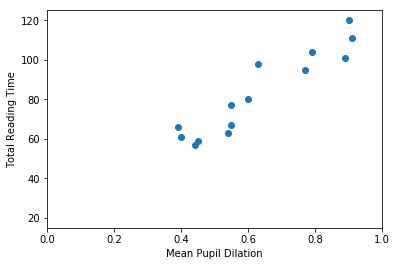It should be quite visible that you can have a good guess (from this data) of one of the values based on the other. That is, that you can guess the Total Reading Time based on the Mean Pupil Dilation

## Formulating the Problem

In this first example, our goal is to find a function that crosses all dots in the graph above. That is, this function should, for the values of mean_pupil_dilation that we know, have the values of total_reading_time in our dataset (or be the closest possible to them). We will also assume that this function is “linear”. That is, we assume that it is possible to find a single straight line that works as a soluton for our problem.

With these assumptions in hand, we can now define this problem in a more formal way. A line can be always described by the function $y = Ax + b$, where the $A$ is referred to as the slope, and $b$ is normally called the intercept (because it is where the line intercepts the $y$-axis when $x = 0$). In our case, the points that we already know about the line are going to help us to decide how this line is supposed to look like. That is:

The equations above came directly from our table above. For one of the participants, when total_reading_time is 66, the mean_pupil_dilation is 0.39. For the next, when the total_reading_time is 61, the mean_pupil_dilation is 0.4. We make the total_reading_time the $y$ of our equation (the value that we want to predict), and it is predicted by a transformation of the mean_pupil_dilation (our $x$).

Of course, you don’t need to be a genius to realize that this system of equations has no solution (that is, that no straight line will actually cross all the points in our graph). So, our goal is to find the best line that gets the closest possible to all points we know. To indicate this in our equations, we insert a variable that stands for the “error”.

Now… this notation is quite cluttered with lots of variables that repeat a lot. People who actually do this normally prefer to write this with matrices. The following equation means exactly the same:

Finally… we often replace the vectors by bold letters and just write it as:

Our goal is, then, for each of the equations above, to find values of $A$ and $b$ such that the $\epsilon_i$ (i.e., the error) associated with that equation is the minimum possible.

## Evaluating a Regression solution

Now… there is a literally infinite number of possible lines, and we need to find a way to evaluate them, that is, decide if we like a certain line more than the others. For this, we probably should use the errors (i.e., the $\boldsymbol{\epsilon}$): lines that have big errors should be discarded, and lines that have low errors should be preferred. Unfortunately, there are several ways to “put together” all the $\epsilon_i$ denoting the errors associated with a given line. One way to “put together” all these $\epsilon_i$ could be summing them all:

However, you might have guessed by the word “naïve” there that this formula has problems. The problem with this formula the following: that, when some points are above and some points are below the line, the errors will “cancel” each other. For example, in the image below, the line does not cross any of the data points, but still produces an $E_{naïve} = 0$. How? The line passes at a distance of exactly 1 from the first five data points, producing a positive error (because the points are above the line) of 1 for each of them; but also passes at a distance of exactly 5 from the sixth data point, producing a negative error (because the point is below the line) of -5. When you sum up everything, you get $E_{naïve} = 1 + 1 + 1 + 1 + 1 - 5 = 0$.

X = [1,2,3,4,5,6]
Y_line = np.array([1,2,3,4,5,6])
Y_dots = np.array([2,3,4,5,6,1])

plt.plot(X, Y_line)
plt.plot(X, Y_dots, 'ro')

[<matplotlib.lines.Line2D at 0x7f1515f17b70>]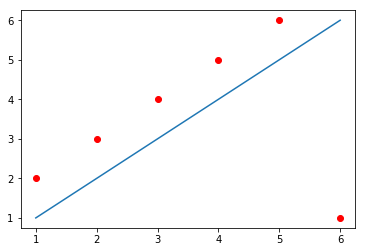One solution to this problem could be to simply use the absolute value of each $\epsilon$ when calculating the error value:

This is a commonly used formula for evaluating the quality of a regression curve. Summing the magnitude of each $\epsilon$ this way is referred to as calculating the $L_1$ norm of the $\epsilon$ vector.

Unfortunately, the absolute-value function is not differentiable everywhere in its domain (that is, the derivative of this function is not defined at the point when $x = 0$ – if you don’t know what derivative or differentiation is, don’t worry, this is not super crucial for understanding the rest). This is not a terrible problem, but we are going to need differentiation later, and a great alternative function that doesn’t have this problem is the $L_2$ norm:

The code below shows each of the alternative errors for the simple example above, where, as we saw, the $E_{naïve} = 0$.

# (Following the example immediately above)

# Calculating the error in a very naive way
print("Error naive: ", np.sum(Y_dots - Y_line))

# Calculating the error using the absolute value of the epsilons:
print("Error L1:    ", np.sum(np.absolute(Y_dots - Y_line)))

# Calculating the error using the absolute value of the epsilons:
print("Error L2:    ", np.sum((Y_dots - Y_line)**2))

Error naive:  0
Error L1:     10
Error L2:     30


This last function (the $E_{L_2}$) is the usual choice for evaluating the Regression line. It is differentiable everywhere, but is not so robust to outliers as the $L_1$ norm.

## Motivating Gradient Descent (a method to find the best line)

In the sections above, we have defined what we want to get: a good line – hopefully, the best one – that (almost) crosses all the points in our dataset. We have also understood how to decide if a line is good or not, based on the errors between the value predicted by the line and the value that appears in our data.

The images below show several possible lines, with an intercept of 0 and slopes 10, 30, 50, 100 and 200. The last graph shows the Sum of Squared Errors (the $L_2$ norm of the error vector $\epsilon$) for each of the lines:

# This is the original data
data_x = mean_pupil_dilation

# Let's create some possible lines
plot_x1 = mean_pupil_dilation
plot_y1 = 10*plot_x1 + 0

plot_x2 = mean_pupil_dilation
plot_y2 = 30*plot_x2 + 0

plot_x3 = mean_pupil_dilation
plot_y3 = 50*plot_x3 + 0

plot_x4 = mean_pupil_dilation
plot_y4 = 100*plot_x4 + 0

plot_x5 = mean_pupil_dilation
plot_y5 = 200*plot_x5 + 0

# Now let's plot these lines, along with the data
def plot_line_and_dots(line, dots, lims):
line_x, line_y = line
dots_x, dots_y = dots
xlim, ylim = lims
plt.plot(line_x, line_y)
plt.plot(dots_x, dots_y, 'o')
plt.xlim(xlim)
plt.ylim(ylim)

plt.figure(figsize=(18, 16), dpi= 200)

plt.subplot(3,2,1)
plot_line_and_dots([plot_x1, plot_y1], [data_x, data_y], [[0,1],[0, 200]])

plt.subplot(3,2,2)
plot_line_and_dots([plot_x2, plot_y2], [data_x, data_y], [[0,1],[0, 200]])

plt.subplot(3,2,3)
plot_line_and_dots([plot_x3, plot_y3], [data_x, data_y], [[0,1],[0, 200]])

plt.subplot(3,2,4)
plot_line_and_dots([plot_x4, plot_y4], [data_x, data_y], [[0,1],[0, 200]])

plt.subplot(3,2,5)
plot_line_and_dots([plot_x5, plot_y5], [data_x, data_y], [[0,1],[0, 200]])

# Finally, in the last plot, let's look at the error between the

plt.subplot(3,2,6)
plt.plot([10, 30, 50, 100, 200],
[sum(squared_errors1),
sum(squared_errors2),
sum(squared_errors3),
sum(squared_errors4),
sum(squared_errors5)], '-ro')

[<matplotlib.lines.Line2D at 0x7f1515d61a90>]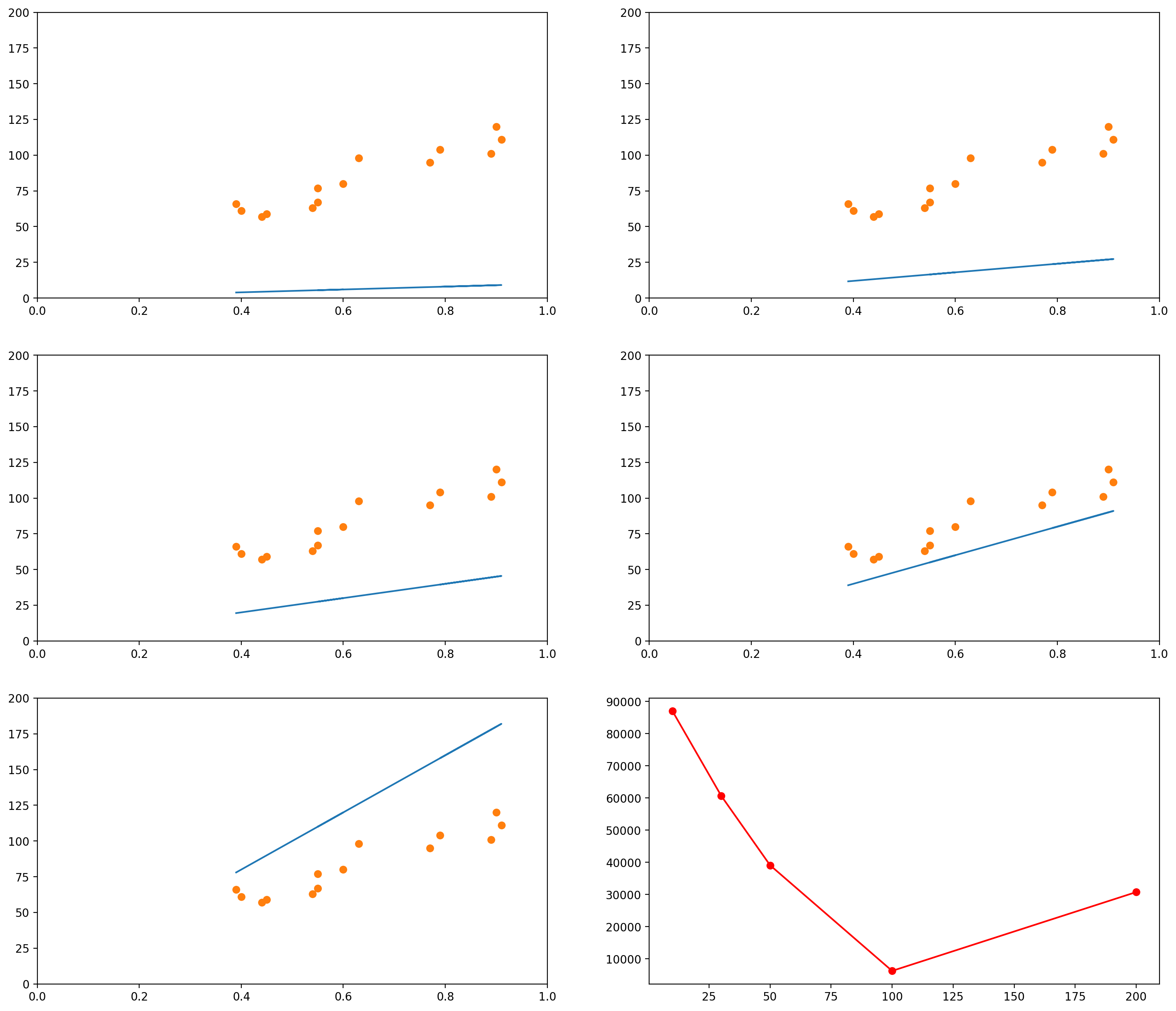As you can see, when the slope is 10 (the first graph, and the leftmost data point in the last graph), the $L_2$ norm of the error vector is very high. As the slope keeps increasing, the error goes on decreasing, until a certain moment (somewhere between the slopes 100 and 200), when it increases again.

We could plot the Sum of Squared errors of many many of these lines, and we would get a function that looks like the following:

# Initialize an empty list
error_l2_norms = []

for i in range(200):
# Gets the y values of the line, given the slope i
plot_y1 = i*plot_x1 + 0
# Calculates the sum of squared errors for all the data points we have
# Inserts the sum in our list
error_l2_norms.append(sum_squared_errors)

# Now we plot the 200 elements of the list, along with the sum of squared errors
plt.plot(range(200), error_l2_norms)

[<matplotlib.lines.Line2D at 0x7f151446d898>]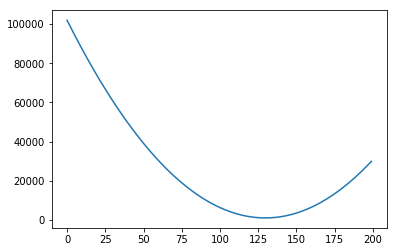Notice that so far we only moved the slope. We could do the same with the intercept. For example, let’s say we fixed our slope in 75. Then we could generate graphs with intercepts, say, 0, 20, 40, 60, 80:

# This is the original data
data_x = mean_pupil_dilation
slope = 75

# Let's create some possible lines
plot_x1 = mean_pupil_dilation
plot_y1 = slope*plot_x1 + 0

plot_x2 = mean_pupil_dilation
plot_y2 = slope*plot_x2 + 20

plot_x3 = mean_pupil_dilation
plot_y3 = slope*plot_x3 + 40

plot_x4 = mean_pupil_dilation
plot_y4 = slope*plot_x4 + 60

plot_x5 = mean_pupil_dilation
plot_y5 = slope*plot_x5 + 80

plt.figure(figsize=(18, 16), dpi= 200)

plt.subplot(3,2,1)
plot_line_and_dots([plot_x1, plot_y1], [data_x, data_y], [[0,1],[0, 200]])

plt.subplot(3,2,2)
plot_line_and_dots([plot_x2, plot_y2], [data_x, data_y], [[0,1],[0, 200]])

plt.subplot(3,2,3)
plot_line_and_dots([plot_x3, plot_y3], [data_x, data_y], [[0,1],[0, 200]])

plt.subplot(3,2,4)
plot_line_and_dots([plot_x4, plot_y4], [data_x, data_y], [[0,1],[0, 200]])

plt.subplot(3,2,5)
plot_line_and_dots([plot_x5, plot_y5], [data_x, data_y], [[0,1],[0, 200]])

# Finally, in the last plot, let's look at the error between the

plt.subplot(3,2,6)
plt.plot([0, 20, 40, 60, 80],
[sum(squared_errors1),
sum(squared_errors2),
sum(squared_errors3),
sum(squared_errors4),
sum(squared_errors5)], '-ro')

[<matplotlib.lines.Line2D at 0x7f151437a4e0>]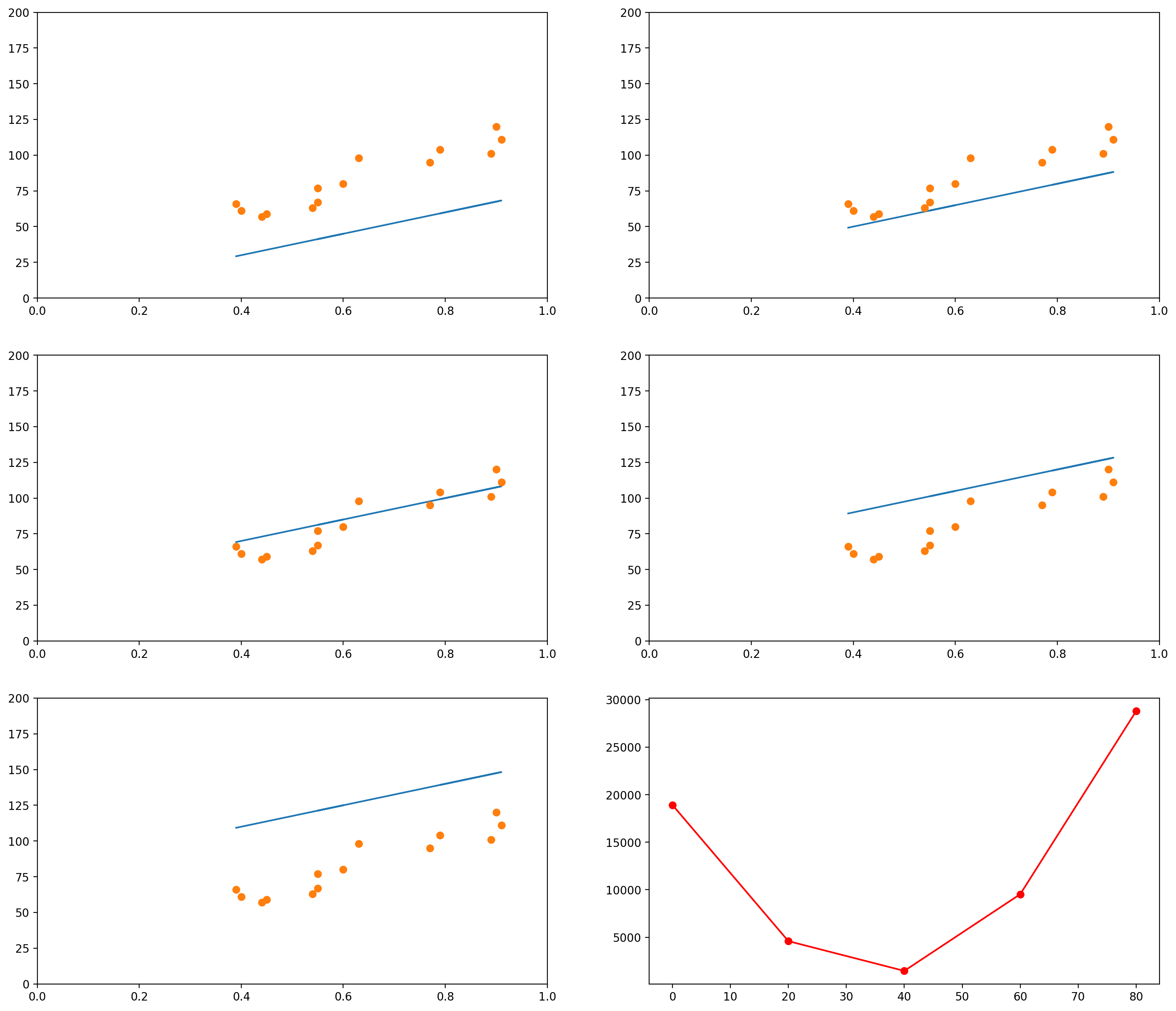Of course, again, we could plot the errors of curves for many other values of intercept:

# Initialize an empty list
error_l2_norms = []
slope = 75

for i in range(100):
# Gets the y values of the line, given the slope i
plot_y1 = slope*plot_x1 + i
# Calculates the sum of squared errors for all the data points we have
# Inserts the sum in our list
error_l2_norms.append(sum_squared_errors)

plt.plot(range(100), error_l2_norms)

[<matplotlib.lines.Line2D at 0x7f1514243198>]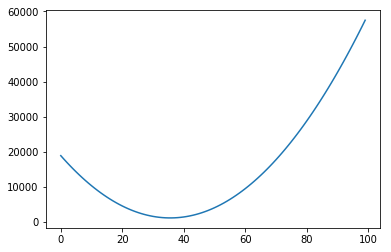In each of the graphs above, we fixed a value for one of the variables (either the intercept or the slope) and iterated through many possible values of the other variable. It is important to notice that, as one of the variables change, the curve for the other variable also changes. In the example above, we had chosen a slope of 75. The example below shows what happens when we use a slope of 200. The graph to the left has an intercept of 0; the graph to the right shows how the error change as the intercept increases from 0 to 100.

slope = 200

# Change the default size of the plotting
plt.figure(figsize=(10, 5), dpi= 200)

plot_x = mean_pupil_dilation
plot_y = slope*plot_x5 + 0
plt.subplot(1,2,1)
plot_line_and_dots([plot_x, plot_y], [data_x, data_y], [[0,1],[0, 200]])

# Initialize an empty list
error_l2_norms = []

for i in range(100):
# Gets the y values of the line, given the slope i
plot_y1 = slope*plot_x1 + i
# Calculates the sum of squared errors for all the data points we have
# Inserts the sum in our list
error_l2_norms.append(sum_squared_errors)

# Now we plot the 100 elements of the list, along with the sum of squared errors
plt.subplot(1,2,2)
plt.plot(range(100), error_l2_norms)

[<matplotlib.lines.Line2D at 0x7f1515de7c18>]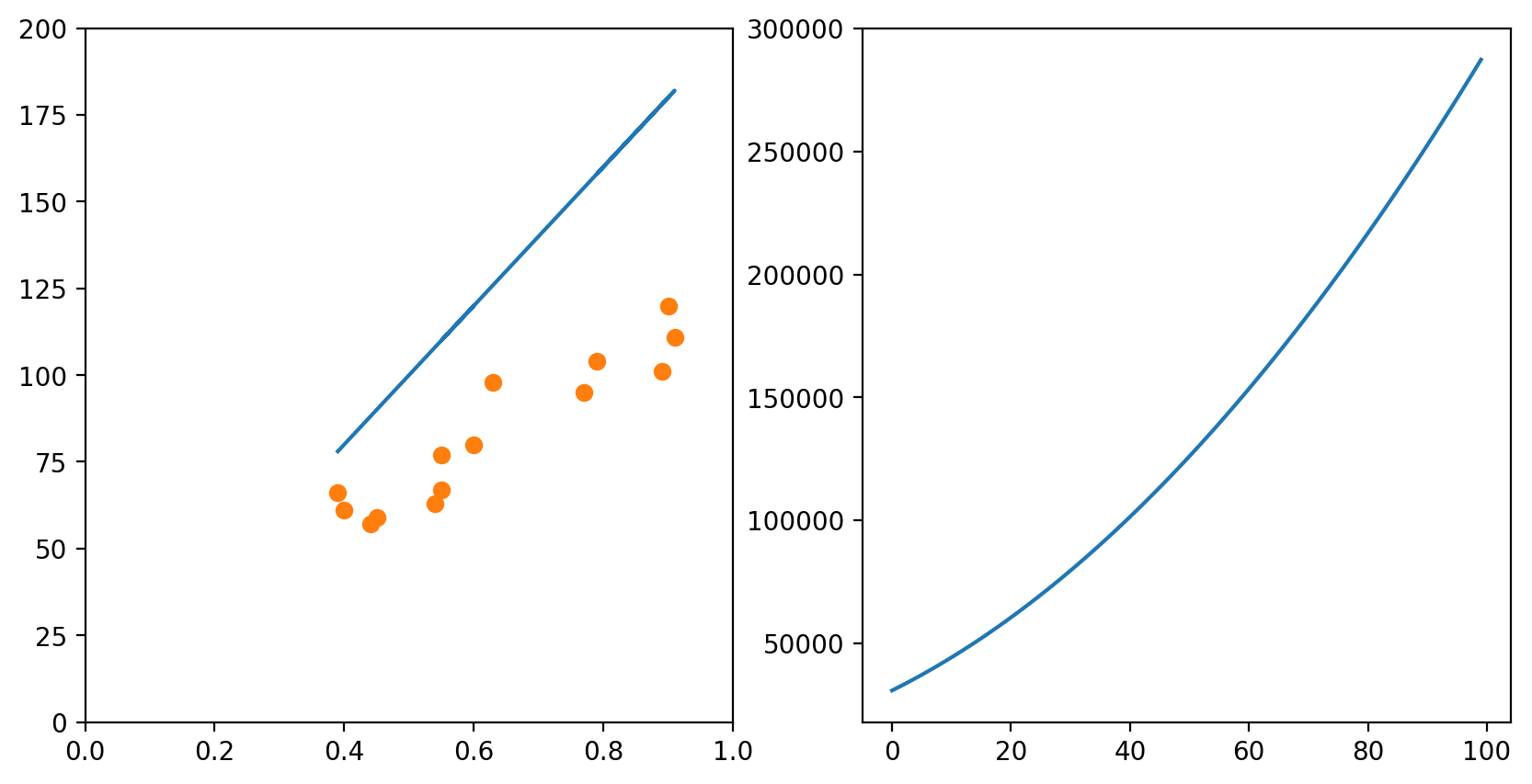Of course, if one had time, one could try all possible combinations of slope and intercept and choose the best one. This would generate a surface in the 3D space:

# Initialize an empty list
error_l2_norms = np.zeros([100, 100])

for i in range(100):
for slope in range(100):
# Gets the y values of the line, given the slope i
plot_y1 = slope*plot_x1 + i
# Calculates the sum of squared errors for all the data points we have
# Inserts the sum in our list
error_l2_norms[i, slope] = sum_squared_errors

X = np.arange(0, 100, 1)
Y = np.arange(0, 100, 1)
X, Y = np.meshgrid(X, Y)
Z = error_l2_norms

fig = plt.figure()
ax = fig.gca(projection='3d')
ax.set_xlabel('Intercept')
ax.set_ylabel('Slope')
ax.set_zlabel('Sum of Squared Errors')
surf = ax.plot_surface(X, Y, Z, cmap=cm.coolwarm, rstride=10, cstride=10)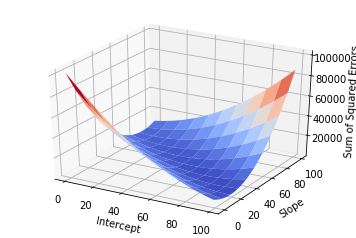But this approach would be too computationally intensive, and if you had more variables it would probably take too long.

To solve this problem in an easy way, we use Gradient Descent. We will first understand the intuition of Gradient Descent, and then I will show the maths.

Using our example above, let’s focus on what Gradient Descent would do if we had the two variables Intercept and Slope and wanted to find the best configuration of Intercept and Slope (i.e., the configuration for which the error is minimum). Gradient Descent would start with any random configuration. Then, given this configuration, it would ask:

• In which direction (and how ‘strongly’) do I need to change my Intercept so that my error would increase?”

In more fancy mathy terms, it would calculate the derivative2 of the error function (the surface plotted above) with respect to the variable Intercept. It would then keep this “direction” in a variable.

At the same time, it would also ask:

• In which direction (and how ‘strongly’) do I need to change my Slope so that my error would increase?”

Again, this is the same as calculating the derivative of the error function with respect to the Slope. It would then also store this “direction” in a variable.

Finally, it would take the current Intercept and Slope and update them using the values it just calculated. But there is a catch: since it calculated the direction in which the error would increase, it updates the two variables in the opposite direction.

### More formally

Now we are ready to understand the formal notation for the algorithm. Remember that our error function is the Sum of Squared Errors, also referred to as the $L_2$-norm of the error vector $\boldsymbol{\epsilon}$, and that this $L_2$-norm is normally written as $| \cdot |_2$3. That is, the $L_2$-norm of $\boldsymbol{\epsilon}$ is normally written $| \boldsymbol{\epsilon} |_2$.

Proceeding, we want to represent the derivative of the error function with respect to the variables Intercept (which we were referring to as $A$) and Slope (which we were referring to as $b$). These derivatives are normally written as

Notice that the error function $| \boldsymbol{\epsilon} |_2$ depends exclusively on these two variables. This leads us to the concept of “Gradient”. The Gradient of the error function is a vector containing the derivative of each of the variables on which it depends. Since $|\boldsymbol{\epsilon}|_2$ depends only on $A$ and $b$, the Gradient of $|\boldsymbol{\epsilon}|_2$ (we represent it by $\nabla |\boldsymbol{\epsilon}|_2$) is the following vector:

After calculating the value of the Gradient, we can just update the value of $A$ and $b$ accordingly:

The $\lambda$ there is the “learning rate”. It is just a number multiplying each of the elements of the Gradient. The idea is that it might make sense to make smaller or bigger jumps if you know you are too close or too far away from a good configuration of parameters.

The Gradient Descent procedure will normally help us find a so-called “local minimum”: a solution that is better than all solutions nearby. Consider, however, the graph below:

# Defines (x,y) coordinates for many points for the curve
x = np.linspace(-30, 10, 200)
y = np.sin(0.5*x) + .3*x + .01*x**2

# Plots the (x,y) coordinates defined above
plt.plot(x, y)

# Plots a red dot at the point x=3
plt.plot(, [np.sin(0.5*3) + .3*3 + .01*3**2], 'ro')

[<matplotlib.lines.Line2D at 0x7f15141414e0>]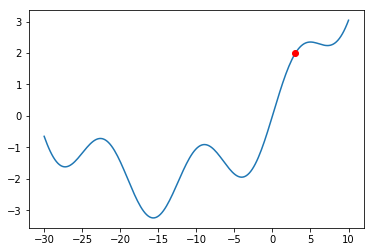What would happen if we were at the red dot and used Gradient Descent to find a solution? The algorithm might get stuck in the local minimum immediately to its right (near $x = -5$), and never manage to find the global minimum (around $x = 15$). You should always keep this in mind when using Gradient Descent.

Even though there might be shortcomings to Gradient Descent, this is the method used in a lot of Machine Learning problems, and this is why I am introducing it here. The problem of Linear Regression is very often a “convex optimization problem”, which means it doesn’t have those local minima above.

## Going beyond 1-dimensional inputs

Of course, the same concepts can be applied when you have more than one variables and you would like to predict the value of another variable. For example, let’s say we now had both the mean_pupil_dilation and the number of fixations (num_fixations below) and we wanted to predict the total_reading_time. In the code below, we will put these values in convenient data structures:

# This was how we had taken the variables separately
mean_pupil_dilation = np.array(data)[:, 1].astype(float)
num_fixations = np.array(data)[:, 3].astype(float)

# We can use the zip() function to put them all together again
# zip() returns a generator... so we use list() to transform it into a list
dilation_fixations = list(zip(mean_pupil_dilation, num_fixations))
print("mean_pupil_dilation", mean_pupil_dilation)
print("--")
print("num_fixations", num_fixations)
print("--")
print("dilation_fixations", dilation_fixations)

mean_pupil_dilation [0.9  0.89 0.79 0.91 0.77 0.63 0.55 0.6  0.55 0.54 0.45 0.44 0.4  0.39]
--
num_fixations [20. 18. 24. 19. 20. 22. 30. 23. 56. 64. 42. 43. 51. 40.]
--
dilation_fixations [(0.9, 20.0), (0.89, 18.0), (0.79, 24.0), (0.91, 19.0), (0.77, 20.0), (0.63, 22.0), (0.55, 30.0), (0.6, 23.0), (0.55, 56.0), (0.54, 64.0), (0.45, 42.0), (0.44, 43.0), (0.4, 51.0), (0.39, 40.0)]


Let’s also plot the data in 3D, to get a notion of how it looks like (it is the same data… even though it might not seem the same at a first glance).

fig = plt.figure()

ax.set_ylabel('Mean Pupil Dilation')
ax.set_zlabel('Number of Fixations')

Text(0.5,0,'Number of Fixations')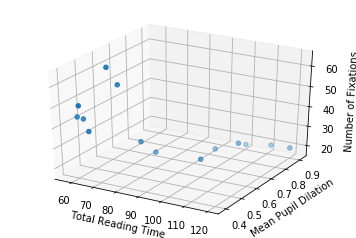So now, with two input dimensions and one output dimension, we don’t only have a line, characterized by a single slope and a single intercept, but a plane, characterized by 3 variables: one intercept and two coefficients.

In the sections above, our line equation looked like this:

Where $A$ was a scalar (a number) and $\mathbf{x}$ was a column vector. That is, the equation looked like this:

Now, instead of having only one $A$, we have two values: $A_1$ and $A_2$. The first value, $A_1$, should be multiplied by the pupil dilation; and the second value, $A_2$, should be multiplied by the number of fixations.

To make this equation function exactly in the same way as before, we can write it like this:

Of course, if you had more variables, you could just add more columns to the $A$ matrix and to the $\mathbf{x}$ matrix. For example, if you had $m$ variables, you would have:

So, putting the numbers in place, remember that we had the following two vectors:

• Pupil dilations: $\begin{bmatrix}0.9 & 0.89 & 0.79 & 0.91 & 0.77 & 0.63 & 0.55 & 0.6 & 0.55 & 0.54 & 0.45 & 0.44 & 0.4 & 0.39\end{bmatrix}$
• Number of fixations: $\begin{bmatrix}20 & 18 & 24 & 19 & 20 & 22 & 30 & 23 & 56 & 64 & 42 & 43 & 51 & 40 \end{bmatrix}$

Then our equation would become:

Just to make it clear, that “$\top$” over the matrix containing our numbers indicates that the matrix was transposed. You could rewrite the equation as:

Then our gradient descent does exactly the same. We first calculate the gradient of the error function, which now is composed by three elements:

And update our variables in the opposite direction:

Or, more generally, if we had $m$ variables,

## Ok… but how do I do Regression in Python? (using sklearn)

We will use the sklearn library in Python to calculate the Linear Regression for us. It receives the input data (the mean_pupil_dilation vector) and the expected output data (the total_reading_time vector). Then it updates its coef_ and intercept_ variables with the slope and intercept, respectively.

(Importantly, because the problem of Linear Regression is quite simple, it is likely not using Gradient Descent in sklearn)

# Adapted from http://scikit-learn.org/stable/modules/linear_model.html

# from sklearn import linear_model

# LinearRegression() returns an object that we will use to do regression
reg = linear_model.LinearRegression()

# Prepare our data
X = np.expand_dims(mean_pupil_dilation, axis=1)

# And print it to the screen
print("X: ", X)
print("Y: ", Y)

# Now we use the reg object to learn the best line
reg.fit(X, Y)

# And show, as output, the slope and intercept of the learnt line
reg.coef_, reg.intercept_

X:  [[0.9 ]
[0.89]
[0.79]
[0.91]
[0.77]
[0.63]
[0.55]
[0.6 ]
[0.55]
[0.54]
[0.45]
[0.44]
[0.4 ]
[0.39]]
Y:  [120. 101. 104. 111.  95.  98.  77.  80.  67.  63.  59.  57.  61.  66.]

(array([106.68664055]), 15.649335484366574)


Now we can just plot the line we found using the intercept and slope we found:

# Now we will plot the data
# Define a line using the slope and intercept that we got from the previous snippet
x = np.linspace(0, 1, 100)
y = reg.coef_ * x + reg.intercept_

# Creates the canvas
fig, axes = plt.subplots()

# Plots the dots

# Plots the line
axes.plot(x, y)

[<matplotlib.lines.Line2D at 0x7f1513efb898>]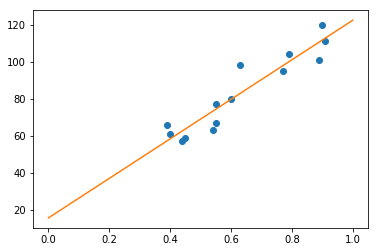# Wrapping Up

Recapitulating, we defined the problem of Regression, defined a (fictitious) dataset on which to base our examples, formulated the problem for one dimension, learned how to evaluate a “solution”, and how this evaluation is used to iteratively find better and better lines (using the Gradient Descent algorithm). Then we expanded the idea for more than one dimension, and finally saw how to do this in Python (actually, we just used a function – which actually probably doesn’t use this method, but, oh, well, the result is what we were looking for).

There is A LOT more to talk about this, but hopefully this was a gentle enough introduction to the topic. In a next post, I intend to cover Logistic Regression. Hopefully, in a third post, I will be able to show how Logistic Regression relates to the artificial neuron.

Very importantly, I think I should mention that this blog post wouldn’t have come into existence if it were not for Kristina Kolesova and Philipp Blandfort, who organized the course of Computational Linguistics in the University along with me, and Shanley Allen, my PhD advisor, who caused us to bring the course into existence. 4

# Footnotes

1. I don’t know exactly how the year is divided in the rest of Germany, but here the semesters start in April and October, and are named Summer and Winter semesters, respectively.

2. This is where we need the derivative, that I spoke about when discussing the possible error functions.

3. I noticed that for some reason the blog is showing only one “|” instead of two. I couldn’t find a way to fix this, so I would like ask you to just consider the “|” and the “||” as the same thing.

4. I didn’t ask them for permission to have them mentioned here (I hope this is not a problem).

# Arrays and Their Multiple Facets

In my first blog post on Convolutions (no need to go read there: this blog post is supposed to be “self-contained”) I discusssed a little about how it would be a good idea to reinterpret the discretized version of the 1D function $f$ as a vector with an infinite number of dimensions. Basically, the only difference between the two ways of viewing this “list of numbers” was that the vector lacked a “reference point”, i.e., the $t$ we had there. Because $f$ was a very nice type of function that was non-zero only for a certain range of $t$’s, we found a way to get this reference point back by dropping the rest of $f$ where $f$ was always zero.

In this blog post, I want to talk about yet another way in which we can look at a vector (and, consequently, at a function $f$). In the next few sections, I will recapitulate the ideas presented in the blog post on Convolutions, explain the other interpretation of vectors, and show how it may be useful when training a classifier.

## Arrays Can Be Reinterpreted As Discrete Functions

Let’s recapitulate what we learned in the previous blog post. In the example, I had a signal $f$ that looked like the following: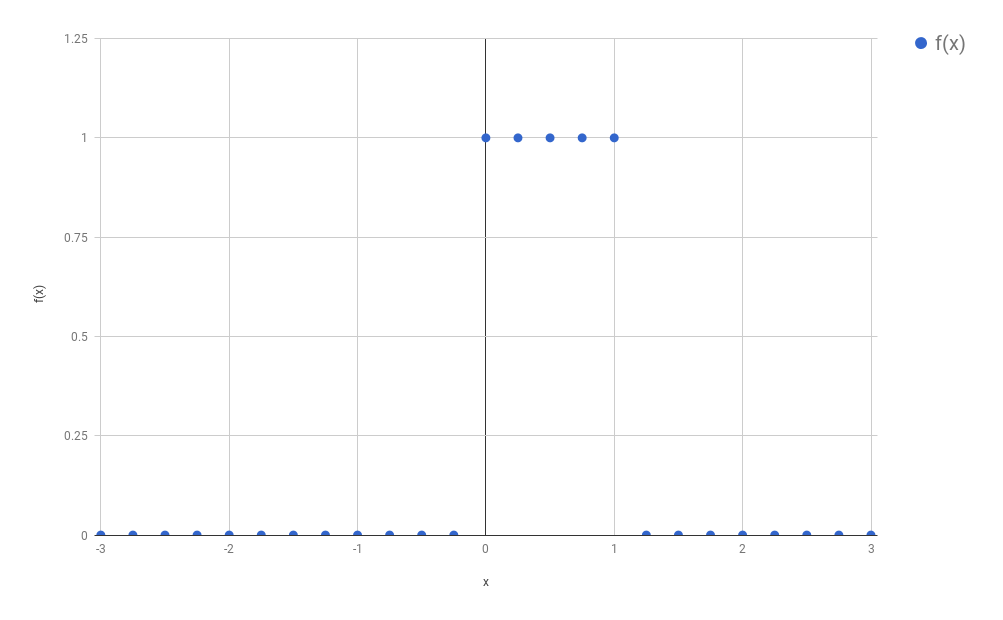Because we wanted to avoid calculating an integral (the calculation of the convolution, which was the problem we wanted to solve, required the solution of an integral), and because we were not dramatically concerned with numeric precision, we concluded it would be a good approximation to just use a discrete version of this signal. We therefore sampled only certain evenly spaced points from this function, and we called this process “discretization”: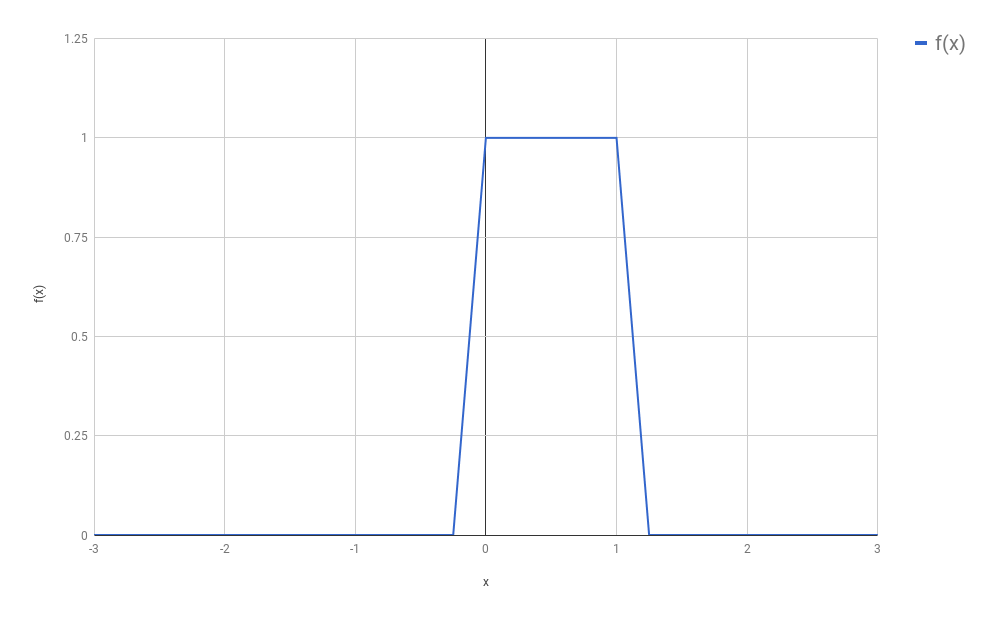(In our original setting, $f$ was a function that turned out to be composed by non-zero values only in a small part of its domain. The rest was only zeros, extending vastly to the right and to the left of that region. This was convenient for our convolutions, and will be convenient too for our discussion below, although most of the ideas presented below are going to still work if we drop this assumption.)

I would like to introduce some names here, so that I can refer to things in a more unambiguous way. Let $f_{discretized}$ be the newly created function, that came into existence after we sampled several points from $f$, all of which are evenly spaced. Additionally, let us call $s$ the space between each sample. For the purposes of this blog post, we will consider we have any arbitrary $s$. It does not really matter how big or small $s$ is, as long as you (as a human being) feel that the new discrete function you are defining resembles well enough (based on your own notion of “enough”) the original $f$. If you choose an $s$ that is too large, you might end up missing all non-zero points of $f$ (or taking only one non-zero point, depending on where you start). If your $s \to 0$, then you have back the continuous function, and your discretization had basically no effect.

Your new function $f_{discretized}$ now could be seen as a vector composed of mostly zeros, except for a small region:

Because this is an infinite array, it is hard to know exactly where it “starts” (or where it “ends”). In the introduction to this post I said this was a “problem”, and we had solved it by dropping the two regions composed exclusively by zeroes:

Of course, we could have retained some of the zeros, if it was for any reason convenient to us. It doesn’t matter much. The main idea here is that we now have a convenient way to represent functions compactly through vectors. This also means that anything that works for vectors (dot products, angles, norms) also should have some interpretation for discrete functions. Think about it!

### Disclaiming Interlude

To say the truth, I don’t think that the lack of a “reference point”, as I said before, is a problem at all. From a “maths” perspective, we could solve this by adopting literally any element as our “start”, and from there we can index all other elements. We could even conveniently choose the element that corresponds to our $t = 0$, and it is almost as if we had $f$ back. Mathematicians are quite used to deal with “infinity”, and these seem quite reasonable ideas.

Other human beings, however, would probably not have the same ease, and our machines have unfortunately a limited amount of memory. We would like to keep in our memory only the things we actually care about… and we don’t care a lot about zeros: they kill any number they multiply with, and work as an identity after the sum.

## Arrays Can Be Reinterpreted As Distributions

It is very likely that, just by reading the heading of this section, you already got everything you need to know. There is no magic insight in here: I just intend to go through the ideas slowly and make it clear why (and, in some ways, how) the heading is true. If you already got it, I would invite you to skip to the next section, that tries to show examples when the multiple facets of vectors are useful. If you stick to me, however, I hope this section may be beneficial.

### What is a Distribution?

When I had a course on Statistics in my Bachelor, it was really bad. At the time of the exam, it seemed I should be much more concerned with how to round the decimal numbers after the comma, than with the actual concepts I was supposed to have learnt. As a consequence, I didn’t understand much of statistics when I started with Machine Learning and it took me a great deal of self-studying to realize some of the things in this blog post.

One of these things was the meaning of the word distribution. This is for me a tricky word, and to be fair I might still miss some of its theoretical details (I just went to Wikipedia, and the article on the topic seems so much more complicated than I’d like it to be). For our purposes here, I will consider a distribution any function that satisfies the following two criteria:

1. It is composed exclusively of positive numbers
2. The area below the curve sums up to 1

(For the avid reader: I am avoiding the word “integral” because I don’t want to bump into “the integral of a point”, that is tricky and unnecessary here)

There is one more important element to be discussed about distributions: any distribution is a function of one of more random variables. These variables represent the thing we are trying to find the probability of. For example, they might be the height of the people in a population, the time people take to read a sentence, or the age of people when they lose their first tooth.

### On Discrete Distributions

(I actually spent a lot of time writing about how continuous distributions could be reinterpreted as vectors, but I have the feeling it was becoming overcomplicated, so I thought I better dedicate one new blog post to my views on continuous distributions)

I believe you should think of Discrete Distributions as the collection of the probabilities that a given random variable assumes any of the values it can assume. For example, let’s say that my random variable $X$ represents the current weather, and that it can be one of the following three possibilities: (1) sunny, (2) cloudy, (3) rainy. Let’s put these three values in a set $\mathcal{X}$, i.e., $\mathcal{X} = \{sunny, cloudy, rainy \}$. Then a probability distribution would tell me all of $P(sunny)$, $P(cloudy)$ and $P(rainy)$. Let’s say that we know the values for these three probabilities:

In that case, it should be easy to conclude that we could represent this probability distribution with the vector $[0.7, 0.2, 0.1]$. Yes! It is this simple! Each one of the outcomes becomes one of the elements of the vector. The ordering is arbitrary. We could have just as well chosen to create a vector $[0.2, 0.7, 0.1]$ from those three values.

### But What If My Vector Does Not Sum Up To 1

It may be too easy to transform a distribution into a vector; but what if I have a vector and would like to transform it into a probability distribution? For example, let’s say that I have some computer program that receives all sorts of data (such as the humidity of the air in several sensors, the temperature, the speed of the wind, etc.) and just outputs scores for how sunny, cloudy or rainy it may be. Imagine that one possible vector of scores is $[101, 379, 44]$. Let’s call it $A$. To facilitate the notation, I would like to be able to call the three elements of $A$ by the value of $X$ they represent. So $A_{sunny} = 101$, $A_{cloudy} = 379$, and $A_{rainy} = 44$. If I wanted to transform $A$ into a distribution, then how should I proceed?

There are actually two common ways of doing this. I’ll start by the naïve way, which is not very common, but could be useful if your values are really almost summing up to 1. (Really… they just need some rounding, and you’d like to make this rounding.) In this case, do it the easy way: just divide each number by the sum of all values in $A$:

This solution would actually work well for our scores. Let’s see how it works in practice:

While this might seem like an intuitive way of doing things, this is normally not the way people transform vectors into probabilities. Why? Notice that this worked well because all our scores were positive. Take a look at what would have happened if our scores were $B = [10, -9, -1]$:

(Ahem)

You could argue that I should, then, instead, just take the absolute values of the scores. This would still not work: the probability $P(X=cloudy)$ would be almost the same as $P(X=sunny)$, even though $-9$ seems much “worse” than $10$ (or even worse than $-1$). Take a look:

So what is the right way? To make things always work, we want to only have positive values in our fractions. What kind of function receives any real number and transforms it into some positive number? You bet well: the exponential! So what we want to do is to pass each element of $B$ (or $A$) through an exponential function. To make things concrete:

The exponential function does amplify a lot the discrepancy between the values (now $sunny$ has probability almost 1), but it is the common way of transforming real numbers into a probability distribution:

This formula goes by the name of softmax and you should totally get super used to it: it appears everywhere in Machine Learning!

## Ok… but… so what? How is this even useful?

More or less at the same time I was writing this blog post, I was preparing some class related to Deep Learning that I was supposed to present at the University of Fribourg (in November/2017). I thought it would be a good idea to introduce the exact same discussion above to the people there. When I reached this part of the lecture, it became actually quite hard to find good reasons why knowing all of the above was useful.

One reason, however, came to my mind, that I liked. If you know that the vector you have is a distribution (i.e., if you are able to interpret it this way), then all of the results you know from Information Theory should automatically apply. Most importantly, the discussion above should be able to justify why you would like to use the Cross-Entropy as a loss function to train your neural network. To make things clearer, let’s say that you were given many images of digits written by hand (like those I referred to in my previous blog post):Now let’s say that you wanted to train a neural network that, given any of these images, would output the “class” that it belongs to. For example, in the image above, the first image is of the “class” 5, the second image is of the the class 0, and so on. If you are used to backpropagation then you would (probably thoughtlessly) write your code using something like the categorical_crossentropy of tflearn (or anything equivalent). This function receives the output of the network (the values “predicted” by the network) and the expected output. This expected output is normally a one-hot encoded vector, i.e., a vector with zeros in all positions, except for the position corresponding to the class of the input, where it should have a 1. In our example, if the first position corresponds to the class 0, then every time we gave a picture of a 0 to the network we would also use, in the call to our loss function, a one-hot encoded vector with a 1 in the first position. If the second position corresponded to the class 1, then every time we gave a picture of a 1 to the network we would also give a one-hot encoded vector with a 1 in the second position to our loss function.

If you look at these two vectors, you will realize that both of them can be interpreted as probability distributions: the “predicted” vector (the vector output by the network) is the output of a softmax layer; and the “one-hot” encoded vector always sums up to 1 (because it has zeros in all positions except one of them). Since both of them are distributions, then we can calculate the cross-entropy $H(expected, predicted)$ as

and this value will be large when the predicted values are very different from the expected ones, which sounds like exactly what we would like to have as a loss function.

## Conclusion

Everything discussed in this blog post was extremely basic. I would have been very thankful, however, if anyone had told me these things before. I hope this will be helpful to people who are starting with Machine Learning.

# A (Very Simple) Introduction to Representation Learning

This blog post is the result of a conversation I had with some friends some time ago. The discussion started when an idea was raised: that the hidden layers of a Neural Network should be called its “memory”. To say the truth, one could think that way, if he wants to think that the network is storing in a “memory” what it has learnt. Still, the way people tend to take it is that these are “latent variables” that the network learnt to extract from the noisy signal that is given to it as input.

This raised the topic of Representation Learning, which I thought I’d discuss a little here. I would like to focus on the task of classification, where a given input must be assigned a certain label $y$. Let’s even simplify things and say that we have a binary classification task, where the label $y$ can be either $0$ or $1$. I’d like to think that I have a dataset $\textbf{x} = {x_1, x_2, x_3, … }$ composed by many inputs $x_i$, where each $x_i$ could be some vector.

Let’s imagine what happens when we start stacking several layers after one another. Even better, let’s see it: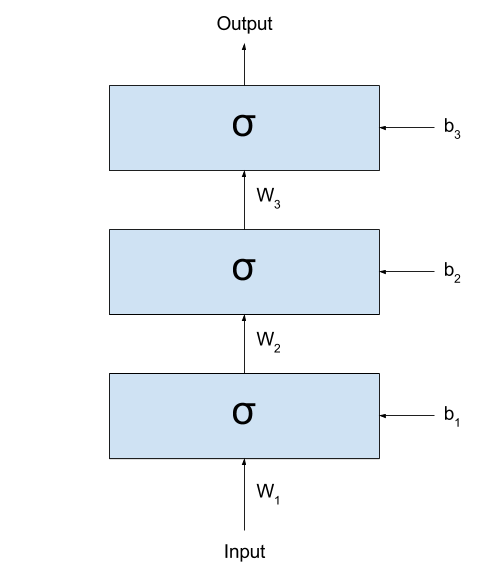If we call the output of the network $y_{prediction}$, we could represent the same network with the following formula:

(I like a lot to look at these formulas. They demystify a lot all the complexity that Neural Networks seem to be built upon.)

As you can see (and as very well discussed in this great Christopher Olah’s blog post), what these networks are doing is basically

• Linearly transforming the input space into some other space (this is done by the multiplication by $W_k$ and sum by $b_y$);
• Non-linearly transforming the input space through the application of the sigmoid function.

Each time these two steps are applied, the input values are more distinctly separated into two groups: those where $y = 0$, and those where $y = 1$. There is, for most $x_i$ in class $y=0$ and $x_j$ is in class $y=1$, the values in $\sigma(W_1 \times x_i + b_1)$ and $\sigma(W_1 \times x_j + b_1)$ will probably be better separable than the raw $x_i$s and $x_j$s. (here, I am using the expression “better separable” very loosely. I hope you get the idea: the values will not necessarily be “farther” from each other, but it will probably be easier to trace a line dividing all elements of the two classes.)

This way, if I treat the inputs as signals, then the input to the next layer could be thought as a cleaned version of the signal of the previous layer. By cleaned version I mean that the output of the previous (lower) layer are “latent variables” extracted from the (potentially) noisy signal used as input.

To make things clearer, I would like to present an example. Imagine I gave you lots of black and white images with digits written by hand: (these are MNIST images. I am linking to an image from Tensorflow. I hope it won’t change the link so soon =) )(to keep the binary classification task, let’s say I want to divide them into “smaller than 5” and “not smaller than 5”.)

The first hidden layer would then receive the raw images, and somehow process them into some (very abstract, hard to understand) activations. If you think well, I could take the entire dataset, pass through the first layer, and generate a new dataset that is the result of applying the first layer to all your images:

After transforming my dataset, I could simply cut the first layer of my network: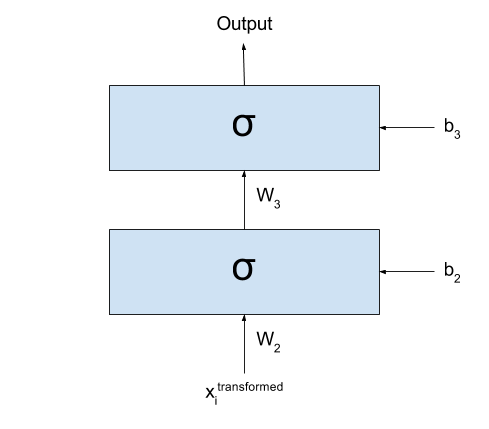Basically what I have now is exactly the same as I had before: all my input data $\textbf{x}$ was transformed into a new dataset $\textbf{x}^{transformed}$ by going through the first layer of my network. I could even forget that my dataset one day were those images and imagine that the dataset for my classification task is actually $\textbf{x}^{transformed}$.

Well, since we are here, what prevents me from repeating this procedure again and again? As we keep doing this multiple times, we would see that the new datasets that we are generating divide the space better and better for our classification problem.

Now, there are many ways in which I can say this, so I’ll say it in all ways I can think of:

• Each new dataset is composed by “latent variables” extracted from the preceding dataset.
• Each new dataset is composed by “features” extracted from the preceding dataset.
• Each new dataset is a new “representation” extracted from the preceding dataset.

Work on learning new representations from the data is interesting because very often some representations extracted from the raw data when performing a certain task may be useful for performing several other tasks. For example, features extracted for doing image classification may be “reused” for, say, Visual Question Answering (where a model has to answer question about an image). This is a vivid area of research, with conferences every year whose sole purpose is discussing the learning of representations!

### However

There is a catch on what I said.

I spent the post saying that, at each step, the layers would separate the data space better and better for the task we are performing. If that is the case, then any network with A LOT of layers would perform very well, right?

But it turns out that only in ~2006 people started managing to train several layers effectively (up to then, many believed that more layers only disturbed the training, instead of helping). Why? The problem is that these same weights that may help in separating the space into a better representation, if badly trained, may end up transforming the input into complete nonsense.

Let’s assume that some of our $W_k$ is so badly trained that, for any given input, it returns something that is completely (REALLY) random (I actually have to stop and think about how possible this might be, but for the sake of the example let’s assume that it is). When out input data crosses that one transformation, it loses all the structure it had. It loses any information, any recoverable piece of actual “usefulness”. From then on, any structure found in the following layers will not reflect the structures found in the input, and we are left hopeless. In fact, we don’t actually even need complete randomness to lose information.. If the “entropy” of the next representation is so high that too many “structures” that were present in the previous layer are transformed into noise, then recovering the information in the subsequent layers may be very hard (sometimes even impossible).

To illustrate how we can lose just some small structures of our data, I will use an example that is related to the meaning of my life: languages. Let’s imagine that there is some dialect of English that makes no difference between two sounds: h and r. So people living in this place say things like This is an a-hey of integers? or I went rome. (incidentally, this is actually not a huge stretch: Brazilians wouldn’t say the second one, but often say the first one. We sometimes really don’t make any difference between the two sounds. But well… we only learn English later, right?)

Now imagine what would happen if a person from this place spoke with another person from, say, the UK. The person from the UK can, most of the times, identify which words are being spoken based on other patterns in the data (for example, he knows that a-hey means array in the sentence above, because he can’t think of any word like a-hey that can go in that context). But what happens if he is talking about a product and the strange-dialect (say, Brazilian) person says:

(1) I hated it as soon as I bought it


Or even, without any context, something like

(2) I saw a hat in the ground


It is simply impossible to distinguish now which of the alternatives is the correct one: both options are right! This is what I mean when I say it is sometimes impossible to recover the information corrupted by some noise.

So what am I trying to say with all this discussion? My point here is that it is not just the introduction of several layers that brings better results, but also the usage of better algorithms for training those layers. This is what changed in ~2006, when some very notable researchers found a good algorithm for initializing each $W_k$ and $b_k$. (This algorithm became eventually known as Greedy Layer-Wise (Pre)Training, although some simply called it by the non-fancified name of “Unsupervised Pretraining”). It had finally become clear the problem were not multiple layers; the problem was elsewhere!

## Conclusion

We went through some Representation Learning, and then discussed the importance of the training process in our networks. Somewhere along with this last discussion, we got an intuition on how noise can corrupt information. The ideas we went through here are very powerful. They are what drives my interest in Deep Learning. I hope you can find them as interesting as I do =)

I would like to thank three friends for having given me the ideas for this post (in alphabetic order to be fair):

• Ayushman Dash: who suggested me to write it.
• Bhupen Chauhan: he started the wondering about the ideas of memory and representation.
• Sidharth Sahu (I’ll add a link for him here soon): a lot of the discussion here are my thoughts about his wonderings during the conversation.

# Convolutions and Neural Networks

In my last blog post, I took you by the hand and guided you through the realm of convolutions. I hope to have made it clear why it makes sense to discretize functions and represent them as vector, and how to calculate the convolution of 1D and 2D vectors.

In this post I want to talk a little about how Image Processing was done in the old times, and show the relation between the procedures performed back then and the kinds of parameters learnt by Convolutional Neural Networks (CNN). In fact, do notice that CNNs have been lurking around for years (LeNet had been introduced in 1998!) before they went viral again in 2012 (with the AlexNet), so, in a way, they are concurrent models to the models described below.

It is hard to tell why Convolutional Neural Networks took so long to become popular. One reason might be that Neural Networks had gone somewhat out of fashion for a while until their revival some years ago. (Hugo Larochelle commented in this TEDx video how there were papers that were rejected simply based on the argument that his approach used Neural Networks.)

Another contributing factor might be that, for a long time, it was a common belief for many people that Neural Networks with many layers were not good (despite the work with LSTMs being done in Europe). They were taken as “hard to train” and empirically many experiments ended up producing better performances for models with just a few (or even only one) layer. CNNs, however, did not suffer from these problems (at least not that much), and the LeNet paper from 1998 had already 5 layers.

But my focus here is not on the architecture of CNNs, nor on their gradient flow or their history. My focus here is on how exactly we can say that the shared weights of a CNN results in a mathematical formulation that is identical to that of the Convolutions that we discussed in the previous post.

## Image Processing

Before I go into the CNNs I want to show why a Convolutional is something that we might want to do to an image. In my previous post, I tried to be as generic as possible, talking about functions and vectors, speaking from a “signal processing” point of view. It turns out that the Image Processing community has its own perspective. So, from now on, I will take $f$ as a 2D image that I want to somehow process, and to $g$ as a kernel.

When we learn math in school, we learn names of several functions that are known to be useful, and somehow represent well parts of the world we live in. Examples of such functions are $log$, $ln$, $sin$, or $tg$. When we are introduced to statistics, we get acquainted to several other names, such as “correlation”, “standard deviation”, “variance”, “mean” or “mode”. The types of kernels used in Image Processing are not different: researchers in the area have found through the years several kernels that are known to perform well different kinds of tasks, such as blurring, edge detection, sharpening, etc. You can find a list of such kernels in the Wikipedia article.

I want to show how a convolution could be used to find the edges of an image. But this time, I don’t want to show formulas; I think some Python code should make things clearer. Let’s say we want to find the borders of the following image of Lenna:The first thing to do is to load the image:

from PIL import Image
img = Image.open('lenna.bmp')


Then I want to create a function to convolve the image with the kernel:

# import numpy as np

def convolve(image, kernel):
# Flips the kernel both left-to-right and up-to-down
kernel = np.fliplr(np.flipud(kernel))

# Transforms the image into something that numpy can process
image_array = np.array(image)

# Initializes the image I want to return
new_image_array = np.zeros(image_array.shape)

# Convolve
for i in range(image_array.shape - kernel.shape):
for j in range(image_array.shape - kernel.shape):
# run_kernel will perform the pointwise multiplication
# followed by sum
new_image_array[i][j] = run_kernel(image_array, kernel, i, j)

# Creates a new Image object
new_image = Image.fromarray(new_image_array)

# Returns both the image as an array, and as an Image object
return new_image_array, new_image


As you can see, I am using numpy to perform the calculations. I expect you not to find it hard to understand the code. It could obviously be written much more efficiently (numpy actually even has a function that performs the convolution anyway), but I wanted to show how the operations we saw in the last blog post can be easily translated into some piece of code.

Now we need to define that run_kernel() function. It calculates the $\odot$ operation between the part of the image that we are interested in and the (already flipped) kernel. This is as simple as:

def run_kernel(image, kernel, pos_x, pos_y):
ret = 0
for i in range(kernel.shape):
for j in range(kernel.shape):
ret += image[pos_x + i][pos_y + j] * kernel[i][j]

return ret


Done! It is that simple!

What we are missing is just the right kernel. If you look at the Wikipedia page you’ll see that there are several kernels usable for Edge detection. I’ll use the third one:

In Python:

new_image_array, new_image = convolve(img, np.array([[-1,-1,-1],[-1,8,-1],[-1,-1,-1]]))
new_image.show()


With this, you should see the following image:Nice, right?

### The Border Problem

If you look carefully at this new image, you’ll see that I’m not running run_kernel() in the last pixels (and then you’ll find some columns of zero pixels at the right of the image, as well as some some rows at the bottom). This has to do with what I called the “Border Problem” in my last post.

It is actually very unclear what should be done in the edges of the Image we are trying to process. The way I have been doing so far, if I calculate a convolution between two $3 \times 3$ matrices, it will give me only one number. If you think well about what the size of the final output would be, you will see that it depends on the kernel size. Let’s assume that our final image has $n$ pixels both horizontally and vertically. For a kernel of size $1 \times 1$ (i.e., just a number), the size of the final image would be the same as the size of the original image If the kernel were $2 \times 2$, then the output would have size $n-1 \times n-1$. For a $3 \times 3$ kernel, it would be $n-2 \times n-2$. You can see how this generalizes to $n-(k+1) \times n-(k+1)$, where $k$ is the size of the kernel.

It would be nice if I could find ways to get a result that had the same size of the input image. The most obvious way to do this is to assume that there are zeros beyond the borders of the images. If you think that the images are signals just like the signals from my previous blog post, you should feel that this is a very reasonable assumption to make. Using this assumptions, you will see three types of convolutions:

• Valid: This is the way I have been doing it so far. We don’t assume any information apart from what we have.

• Full: This is the case where we assume there are lots of zeros beyond that the edge of the original image. This way, if we were given the image $f$ below, then it would be “transformed” into the $f_{transformed}$ below before convolving. The number of new rows/columns introduced depends on the size of the kernel. As I said, this should make sense from the perspective of signal processing I described in my previous post. (if this is not clear enough, you are welcome to take a look at this amazing explanation I found in Stack Overflow)

• Same: This is a little trickier. It also assume zeros around the image, but only as much as needed to return an output that has the exact same size as the input image. I tend to find it hard to visualize, but I found that this image helped a lot.

## Relation to Convolutional Neural Networks

Ok… so I think we covered everything there was to cover about Convolutions. Now I just need to answer: how do they relate to CNNs?

Remember how the convolutions are being calculated: for a given point in “time”, we multiply the values of both matrices pointwise and then sum them all. Now… remember how the connections of the Convolutional Layer are organized: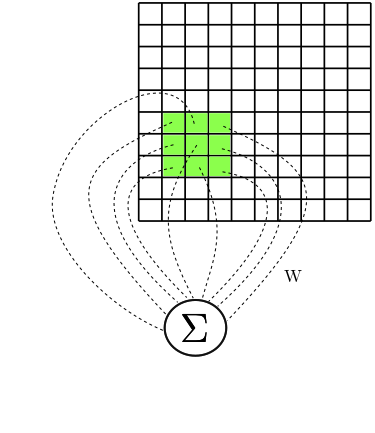Let’s look at one neuron individually. I’d like to call it $a$. It has access to a certain rectangular part of the image. Let’s represent the values of this rectangular part by $A$. So, for example, $A_{0,0}$ represents the element in the leftmost and topmost corner of that rectangular part of the image that our neuron $a$ has access to.

Now, let’s say that $W$ is a matrix with the weights corresponding to the connections between $a$ and the values in $A$. Then the input to $a$ is calculated as

Doesn’t this look a lot like the $\odot$ operation from our kernels? It looks a lot like I am running run_kernel() giving as input the subimage $A$ and the kernel $W$.

Now, let’s focus on another neuron, $b$, and again use a new matrix $B$ to represent the rectangular part of the image that our second neuron has access to. (I hope you see where this is going.) Again, let $V$ denote a matrix composed of the weights of the connections between $b$ and $B$. Then, again, the input to $b$ is calculated as

Again, it looks a lot like I just calculated $B \odot V$, doesn’t it?

If this is hard to see with the formulas, the following image should help a little. It shows the subimages $A$ and $B$, and the connections $W$ and $V$, and how the values are summed when given as input to our neurons $a$ and $b$: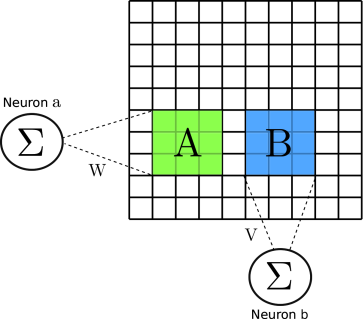Ok, so now you know that the Convolutional layer is running our $\odot$ operation on small subparts of the image. There is just one last point to be made: Convolutional Neural Networks use shared weights. This means the $W = V$! And this also means that the kernel $W$ (or $V$) is always the same for whichever neuron you choose. This means that if I chose at random any new neuron $c$ to inspect (and defined $C$ as the matrix corresponding to the rectangular part of the input image that $c$ has access to), then the calculation that I would perform would still be

(because, as I said $W = V$!)

In summary, this means that the operation these layers are performing is identical to a Convolution!

### Why do we want CNNs?

Now you could ask me: ok, the Image Processing community knows all of these kernels that do magic with my images. Why would I care to have a complex architecture that ends up doing exactly the same kind of thing?

The answer I am going to give is simple, but has huge implications. So far, the Image Processing community had to use their knowledge about how real images generally look like and burn a lot of their own neurons (I mean, figuratively) to generate kernels that somehow fit the problems they were trying to solve. So, if they wanted to find characteristics in the images that would help them to solve the problem they were trying to solve, they had to manually invent kernels that they deemed useful for their task. Many of these kernels followed some patterns/constraints of, e.g., summing up to 1, so that the values of the output image wouldn’t saturate. These patterns somehow limited the types kernels that one could invent, and it was very unintuitive to create anything following different patterns.

But what if, instead of creating kernels by hand (and being bound by constraints, and by our intuition) we could just give a lot of data to a statistical model and just hope that it learns something useful in the end? This is exactly what Convolutional Neural Networks are for. The kernels that are learnt by the CNN are generally not very intuitive, and probably no human would have easily guessed that they are useful for the tasks that these networks are trying to solve (be it classification, of segmentation, or whatever). Still, they have shown great results, and (I would go so far as to say that) the times of “handcrafted feature engineering” are probably over.

## Bonus: Shifting a Signal

Before concluding this blog post, I want to show how convolutions can be unexpectedly useful to perform some seemingly unrelated task: the shifting of a signal. I learnt this in the Neural Turing Machines paper and found it a very elegant way of solving the problem. In this section, I’ll go back to my old notation and refer to the 1D signal $f$. Let’s say it is a discrete signals represented by the following vector:

Now let’s say I want to shift all elements of $f$ to the right. How would I do? One way to do it could be to make a “same” convolution of $f$ with a function $g = [1,0,0]$. Let’s see how this would work.

(here, I am taking $t=0$ is when the first element of $f$ is aligned with the element in the center of $g$)

And what if I wanted to shift it to the left? Just use a different function $g = [0, 0, 1]$:

This example should also give an intuition of how convolutions are a good way of processing signals. In the case of the Neural Turing Machines, instead of shifting the signals so “binarily” to the right or to the left, they allow continuous values to the positions of $g$. For example, $g$ could be anything like $[0.8, 0.1, 0.1]$. In that case, most of the signal would be shifted, but part of the information would remain “spread” (“blurred”) through other positions of the signal. While this may be unintuitive, we have seen how unintuitive things may actually be useful for solving some tasks.

## Conclusion

I hope to have given a good notion of how CNNs relate to the convolutions we saw in the previous post. My hope is that this will provide a good intuition for how convolutions can be used for other Machine Learning architectures, and allow you to think of convolutions as just some other tool that you can use to solve your problems. As you can see, all of this is very simple, but I wish someone had shown me these ideas when I started learning, instead of having to learn them all by myself. I hope this post makes it easy to extend architectures based on convolutions in a way that is sensible taking into account everything discussed here.

# What are Convolutions?

For quite some time already I have been wanting to write this blog post. A little more than one year ago I got acquainted to Convolutional Neural Networks, and it didn’t immediately strike me why they are called that way. I eventually read this blog post that helped a lot to clarify things; but I thought I could try to give more details on what exactly is meant when one says “Convolution” here.

This blog post builds upon the description given there, so, if you still didn’t read that, stop reading this and go there take a look at that blog post. I may overlap some of the discussions here with the discussions there.

In the sections that follow, I’ll introduce convolutions (actually, I’ll let Kahn Academy do that for me), then introduce a procedure to calculate it, motivate a discussion about discrete convolutions, show why it makes sense to represent the convolving functions as vectors and extend the definition to the 2D space. The next blog post will explain why these are useful for signal processing and what is their relation with Convolutional Neural Networks.

## Convolutions

Convolutions are a very common operation in signal processing. While the colah’s blog post presents it in a more abstract/intuitive statistical way, I find that a more gore calculus-driven introduction from Kahn Academy might help you realize that the concept is just an integral:

In this Kahn Academy video, Sal found a closed formula for the convolution by solving the integral. Given that a convolution is an integral, you might consider that it represents the area below some curve. But what curve exactly? I’ll discuss more about it in the next section. For now, what is worth is to understand that there several ways in which you can think of convolutions, and it might help a lot if you allow yourself to switch views at different points in time.

## A concrete example

If you go to the Wikipedia article on convolutions, you may find the following two (awesome) images: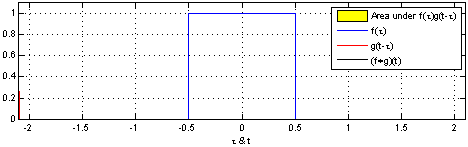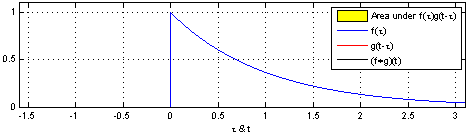What these images are saying is that you can calculate the value of the convolution $f \ast g$ at the point $t$ by following a very simple procedure. I’ll define two functions $f$ and $g$ to make the steps easier to follow. Let

and

Here we have the two curves: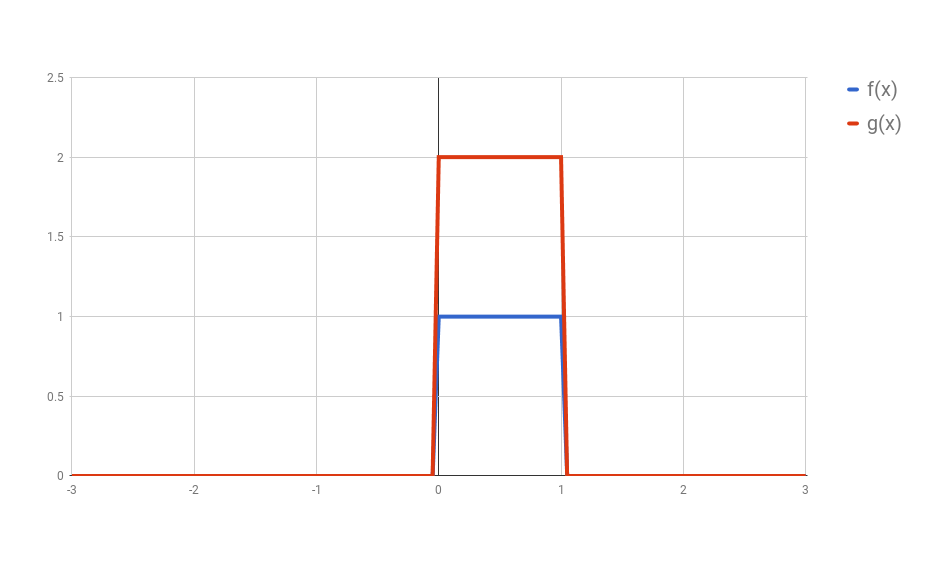(I used Google Spreadsheets to do this, so you’ll notice the lines are not exact, but you should be able to get the idea)

First: flip $g$ horizontally (i.e., $g(x) <- g(-x)$). Let’s give the flipped $g$ a name, say $g’$. (if you don’t flip $g$, then what you are calculating has actually the name of “cross-correlation”, and is simply another typical operation in signal processing.).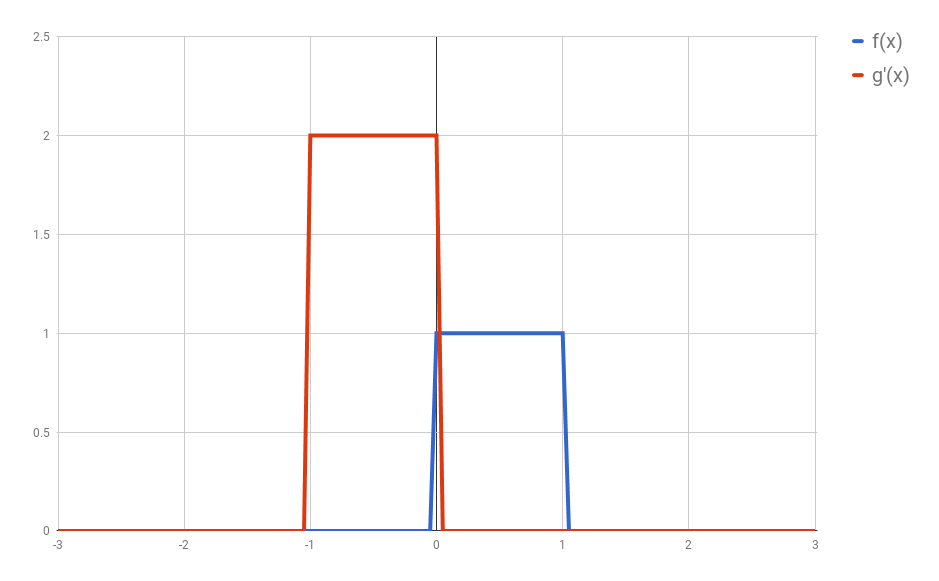Second: shift $g’$ horizontally by $t$ units. If $t$ is positive, then $g’$ will be shifted to the right; otherwise, it will be shifted to the left. For our example, let’s say that $t=0.3$. I’ll call this function $g_{shifted}’$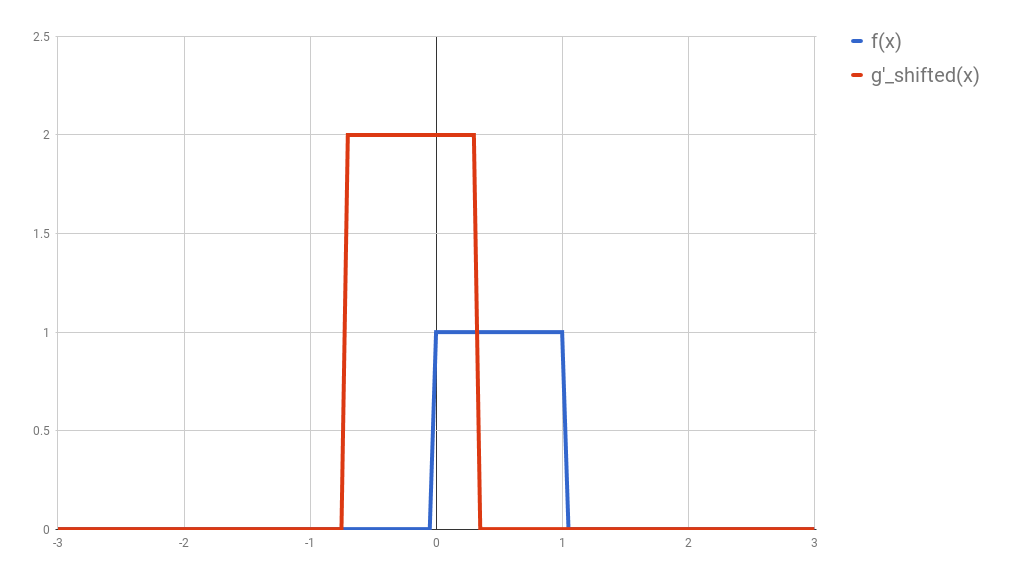Third: this is the step where the problems arise. Now what you want is actually multiply the two curves are each point between $-\infty$ and $+\infty$ and calculate the area below the curve that this multiplication will form. Let’s assume that the functions are zero most of the time (just like in our example), and non-zero only in a small section of their domain. Because we are multiplying the two values, we only care about the values where both functions are not 0. In all other cases, the integral will be 0 anyway. Let’s assume that both functions are non-zero only in an interval $[a, b]$. In this case, our problem reduces to calculating the integral of the multiplication of $f$ and $g_{shifted}’$ inside that interval. Now it could still be a challenge to calculate the integral of the $g_{shifted}’$ and “f” in that interval.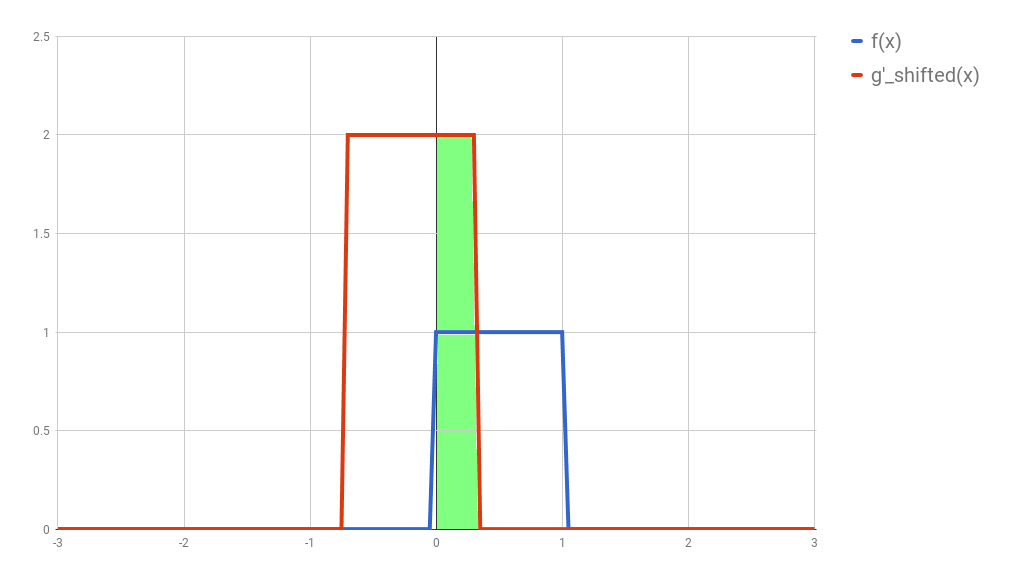(While searching for a way to understand this procedure, I came across this very nice demo. In it you can define your own functions and play arround to find out how the convolution is going to be.)

The problem with continuous convolutions is that we would have to actually calculate an integral. But what if our function were actually “discrete”? Fortunately for us, most applications on Image Processing require discrete signals, and for our purposes it would be perfectly ok to discretize these continuous signals.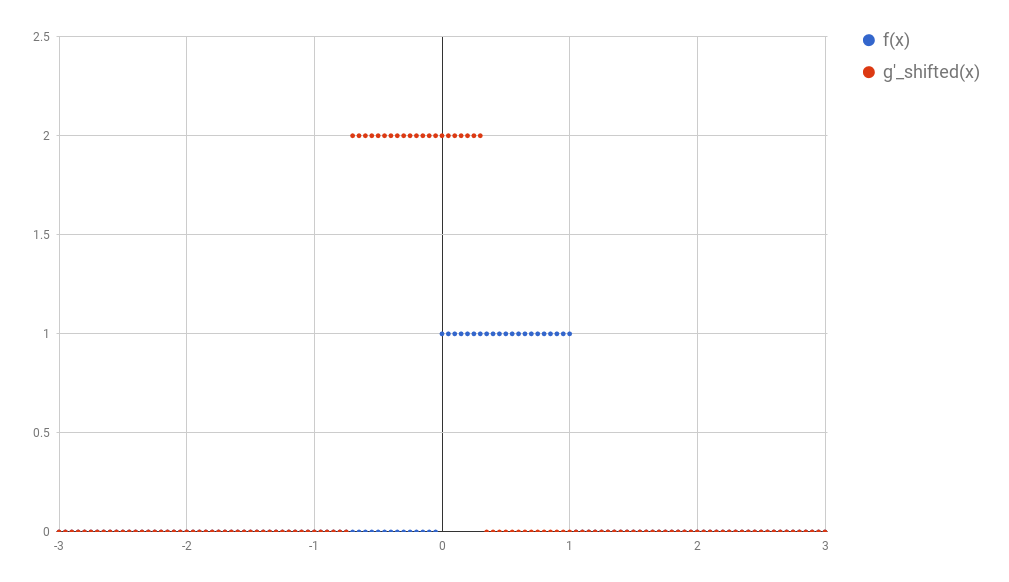After discretization, All the concepts we have discussed so far would follow the same logic. Now, instead of an integral we now have a sum. So, given the interval $[a, b]$, we could calculate the convolution as

And fortunately this sum is easy to calculate.

Note: the avid reader may notice that the integral of an interval spanning only a point should have been 0 (and therefore the convolution should always have become 0 after the discretization). The reason why this does not work has to do with the dirac delta function, and I won’t go into many details here. You can just assume that the discretized version of the signal is a sum of dirac delta functions.

In the example above I discretized the functions using 1 point for each 0.05 step in $x$. This would make the discussion below very hard to understand. So, to make things simpler, in all the text that follows I’ll use steps of 0.25 instead. The image below shows how the original functions $f$ and $g$ would look like discretized this way.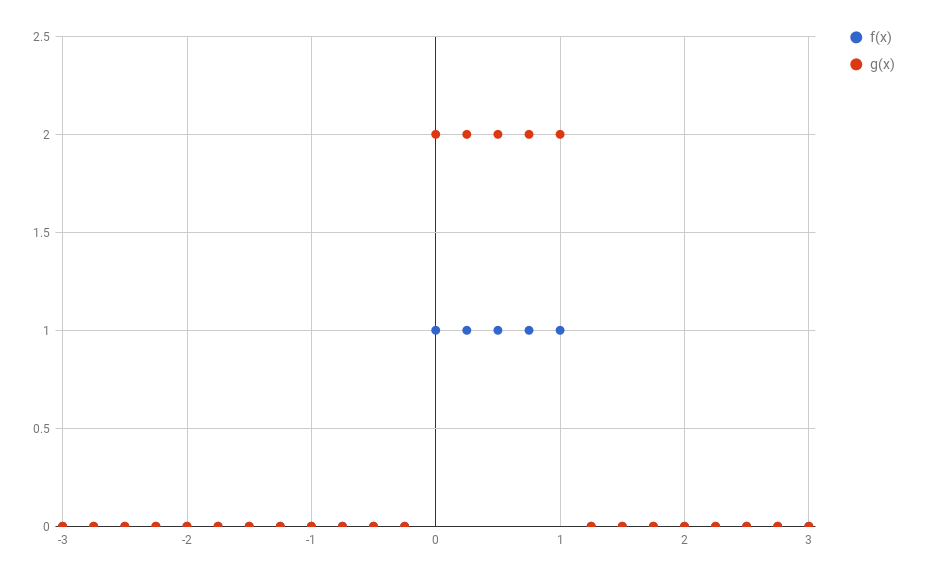## 1D discrete convolutions

It turns out that the functions $f$ and $g$ used in convolutions are in reality most of the times composed almost entirely by zeros (as assumed before). This allows for a much more compact representation of the functions as a vector of values. For example, $f$ and $g$ could be represented as:

$f = [\dots 0, 0, 1, 1, 1, 1, 0, 0, \dots] \\ g = [\dots 0, 0, 2, 2, 2, 2, 0, 0, \dots] \\$ (Of course, the number of 1 and 2 depends on how the discretization was performed)

Now let’s say I’d like to calculate the value of the convolution between $f$ and $g$ at the point $t =$some coordinate. It is hard to point the exact place, so I’ll make the place bold:

$f = [\dots 0, 0, 1, 1, \textbf{1}, 1, 0, 0, \dots] \\$ (For future reference, I’ll call this position $t=2$)

The way to calculate it is just the same:

• Flip $g$ (but it has no effect here, because $g$ is symmetric anyway);

• Move $g$ horizontally by $t$: this is a little abstract here; but if we align the $f$ and $g$ the way they were initially aligned, then we should get:

• Multiply all elements position by position and sum them all.

You might have noticed how these operations may resemble dot-products. You could have implemented them as:

This way, if you wanted to calculate the convolution for many different values of $t$, you could just keep shifting the vector $g$.

Unfortunately, these are still vectors with an infinite number of dimensions, which are hard to store in our limited storage computers. It is worth noting that very often the functions $f$ and $g$ for which we want to calculate a convolution are 0 most of the time. Since we know that the result of the convolution in these regions will be zero, we can just drop all of the zeros:

% (As you can see, I kept some of the zeros. I could have removed them. It was my choice)

And congratulations, we just arrived in a very compact representation of our functions.

Note: The entire discussion so far supposed that we would keep $f$ still and always transform $g$ according to our three steps to calculate the convolutions. It turns out that convolutions are commutative, and therefore the entire procedure would have also worked by holding $g$ still and changing $f$ in the same way. (Incidentally, they are also associative)

## But what does all of this mean?

When I started talking about convolutions, I said that they are used a lot in the context of signal processing. It might be a good idea to forget that these vectors are functions for a while and consider them signals. (this video might help to convince you that this is a sensible idea.) In that case, what a convolution is doing is taking two signals as input and generating a new one based on those two. How the new signal looks like depends on where both signals are non-zero. In the next blog post you’ll see how this can be used in meaningful ways, like finding borders in an image, blurring an image, or even shifting a signal in a certain direction.

Most importantly, convolutions are a very simple operation (composed of sums and multiplications that can be done parallely), which can be easily implemented in hardware. They are a great tool to have in hand when solving difficult problems.

## 2D Convolutions

It shouldn’t be a big leap to extend these concepts to the 2D space.

Let us skip all the discussion about continuous functions and vectors with infinitely many elements and consider our current state: functions $f$ and $g$ are represented as small vectors, and we want to calculate the convolution of those two functions (vectors) at any point $t$. If we now define new $f$ and $g$ in a 2D space, then we can represent them as matrices. For example, if we now redefine $f$ as

and rediscretize it in the same way we did before, then we would get a matrix that looks something like:

(Do not forget: I was the one who decided to keep a border with zeros. I could have left many more columns and rows with zeros in the borders. This may seem irrelevant for now, but will be useful when we discuss kernels in the next blog post.)

Let us define a new $g$, that after discretization looks like the following:

How would the convolution then be calculated? Same steps:

• Flip the matrix $g$ (both horizontally and vertically), generating $g’$.

• Shift $g’$ (according to the place where you want to evaluate the convolution). Basically, you want to align $g’$ with some part of $f$.

• Multiply the aligned elements and sum their result.

### An example calculated by hand

Before concluding this blog post, I want to calculate an example by hand. If you did not understand everything so far, this should clarify whatever is missing. Let’s define two new functions $f$ and $g$, that, after discretization and “vectorization”, become the following matrices:

If you think of $f$ as an image, you might interpret it as two diagonal lines (the values with 6) surrounded by some “shade” (the values with 3). The function $g$, on the other hand, is hard to interpret. I chose a very asymmetric matrix to show how the flipping (the first step in our calculation) affects the final values in $g$.

Let’s calculate $(f \ast g)(0,0)$. First is to flip $g$ to create $g’$:

Then we align the matrix $g’$ with the part of $f$ that corresponds to position $(0,0)$. This part might cause some confusion. Where exactly is $(0,0)$? There is no actual “right answer” to where this point should be after discretization, and we don’t have the original function formula to help us find out. I’ll call this “the border problem” and refer to it in the next blog post. For now, I’ll just align with the points “we know” and forget about any zeros that might lurk beyond the border of the matrix representing $f$. This will give us a so-called “valid” convolution.

Finally, we need to multiply each element pointwise and sum all of the results. To make things clearer, if $A$ and $B$ denoted the two matrices of same size that we now have, then what we want to do is:

Where I am representing this “pointwise multiplication followed by sum” by the operator $\odot$. In our specific case, we get:

Easy, right?

Now to calculate $(f \ast g)(1,0)$ we just move the matrix $g$ to the right, aligning it with the next submatrix of $f$:

And the other two elements are calculated the same way:

Resulting in the final matrix:

## Conclusions

In this blog post I expect to have given you a very intuitive understanding of how convolutions are calculated and a notion of what they are doing. It should help you to make the connection between all those integrals you find in Kahn Academy or Wikipedia and the discrete convolution operation you see in some Neural Networks. If none of this still happened, the examples of the next blog post will definitely help you to realize what is going on.

I had not planned for this blog post to become so long. In the next blog post I’ll show applications of convolutions from the image processing field, and how they connect to Convolutional Neural Networks. As a bonus, I want to show a very elegant application of convolutions from the Neural Turing Machines.

Stay tuned =)

UPDATE: Thanks to Fotini Simistira for pointing some mistakes in my calculations.